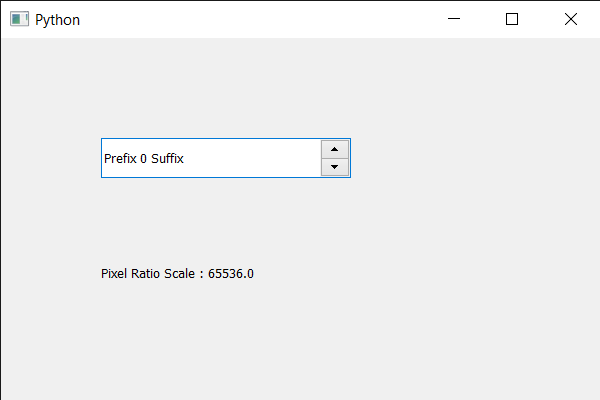Skip to content
Related Articles
PyQt5 QSpinBox – Getting Pixel Ratio Scale
• Last Updated : 19 May, 2020

In this article we will see how we can get the pixel ratio scale for the spin box. The device pixel ratio is the ratio between physical pixels and logical pixels of the spin box. Pixel ratio scale is the type of variable measurement scale which is quantitative in nature. Ratio scale allows any researcher to compare the intervals or differences.

In order to do this we use devicePixelRatioFScale method

Syntax : spin_box.devicePixelRatioFScale()

Argument : It takes no argument

Return : It returns float

Below is the implementation

 `# importing libraries``from` `PyQt5.QtWidgets ``import` `*` `from` `PyQt5 ``import` `QtCore, QtGui``from` `PyQt5.QtGui ``import` `*` `from` `PyQt5.QtCore ``import` `*` `import` `sys`` ` ` ` `class` `Window(QMainWindow):`` ` `    ``def` `__init__(``self``):``        ``super``().__init__()`` ` `        ``# setting title``        ``self``.setWindowTitle(``"Python "``)`` ` `        ``# setting geometry``        ``self``.setGeometry(``100``, ``100``, ``600``, ``400``)`` ` `        ``# calling method``        ``self``.UiComponents()`` ` `        ``# showing all the widgets``        ``self``.show()`` ` `        ``# method for widgets``    ``def` `UiComponents(``self``):``        ``# creating spin box``        ``self``.spin ``=` `QSpinBox(``self``)`` ` `        ``# setting geometry to spin box``        ``self``.spin.setGeometry(``100``, ``100``, ``250``, ``40``)`` ` `        ``# setting range to the spin box``        ``self``.spin.setRange(``0``, ``999999``)`` ` `        ``# setting prefix to spin``        ``self``.spin.setPrefix(``"Prefix "``)`` ` `        ``# setting suffix to spin``        ``self``.spin.setSuffix(``" Suffix"``)`` ` `        ``# getting pixel ratio scale``        ``pixel_ratio_scale ``=` `self``.spin.devicePixelRatioFScale()`` ` `        ``# creating label``        ``label ``=` `QLabel(``self``)`` ` `        ``# setting geometry to the label``        ``label.setGeometry(``100``, ``200``, ``200``, ``70``)`` ` `        ``# making it multi line label``        ``label.setWordWrap(``True``)`` ` `        ``# setting text to the label``        ``label.setText(``"Pixel Ratio Scale : "` `+` `str``(pixel_ratio_scale))`` ` `# create pyqt5 app``App ``=` `QApplication(sys.argv)`` ` `# create the instance of our Window``window ``=` `Window()``window.spin.setFocus()``# start the app``sys.exit(App.``exec``())`

Output :Attention geek! Strengthen your foundations with the Python Programming Foundation Course and learn the basics.

To begin with, your interview preparations Enhance your Data Structures concepts with the Python DS Course. And to begin with your Machine Learning Journey, join the Machine Learning – Basic Level Course

My Personal Notes arrow_drop_up